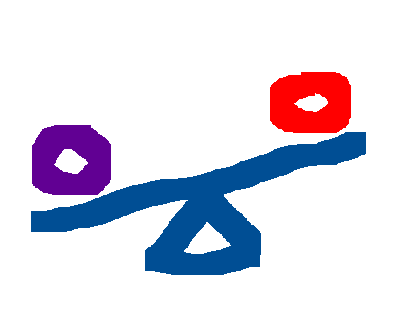# Equation Tutor

Learn algebra by solving equations interactively

Equation:
Puzzle:

Equation Tutor is an interactive teaching tool to help students practice solving equations.

## How to Use Equation Tutor

In Equation Tutor, you are given algebra equations to solve.

### Do to both sides

Under the puzzle diagram, there is a box labeled "Do to both sides". In this box, you can enter operations to solve the equation.

Example operations to "do to both sides":

• -5 (Subtract 5 from both sides)
• -x (Take an 'x' away from both sides)
• /3 (Divide both sides by 3)

### Feedback (Age 13+)

Please click the button at the bottom-right of the page (you should see a question-mark '?' bubble) to send your feedback. Thanks!

Training